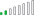cancel
Showing results for
Did you mean:Frequent Visitor

## Wrong Sum totals when using a SUM measure from two different Tables

Dear All PBI Users,

I am getting the wrong columnb totals when i create a measure between two different tables. See Attached picture. How do i solve this.

The formula i have used in shown in the formula bar.

Kindly this is urgent if someone could assist at the soonest

Thanks1 ACCEPTED SOLUTIONResolver II

Please use the following DAX :

MeasureName = SUMX ( TableName, CALCULATE ( SUM(TableName[ColumnName1]) * SUM(TableName[ColumnName2 ]) ) )

This should work...

Thanks,

Himanshu

2 REPLIES 2Resolver II

Please use the following DAX :

MeasureName = SUMX ( TableName, CALCULATE ( SUM(TableName[ColumnName1]) * SUM(TableName[ColumnName2 ]) ) )

This should work...

Thanks,

HimanshuFrequent Visitor

This works perfect.

Thanks Himanshu.